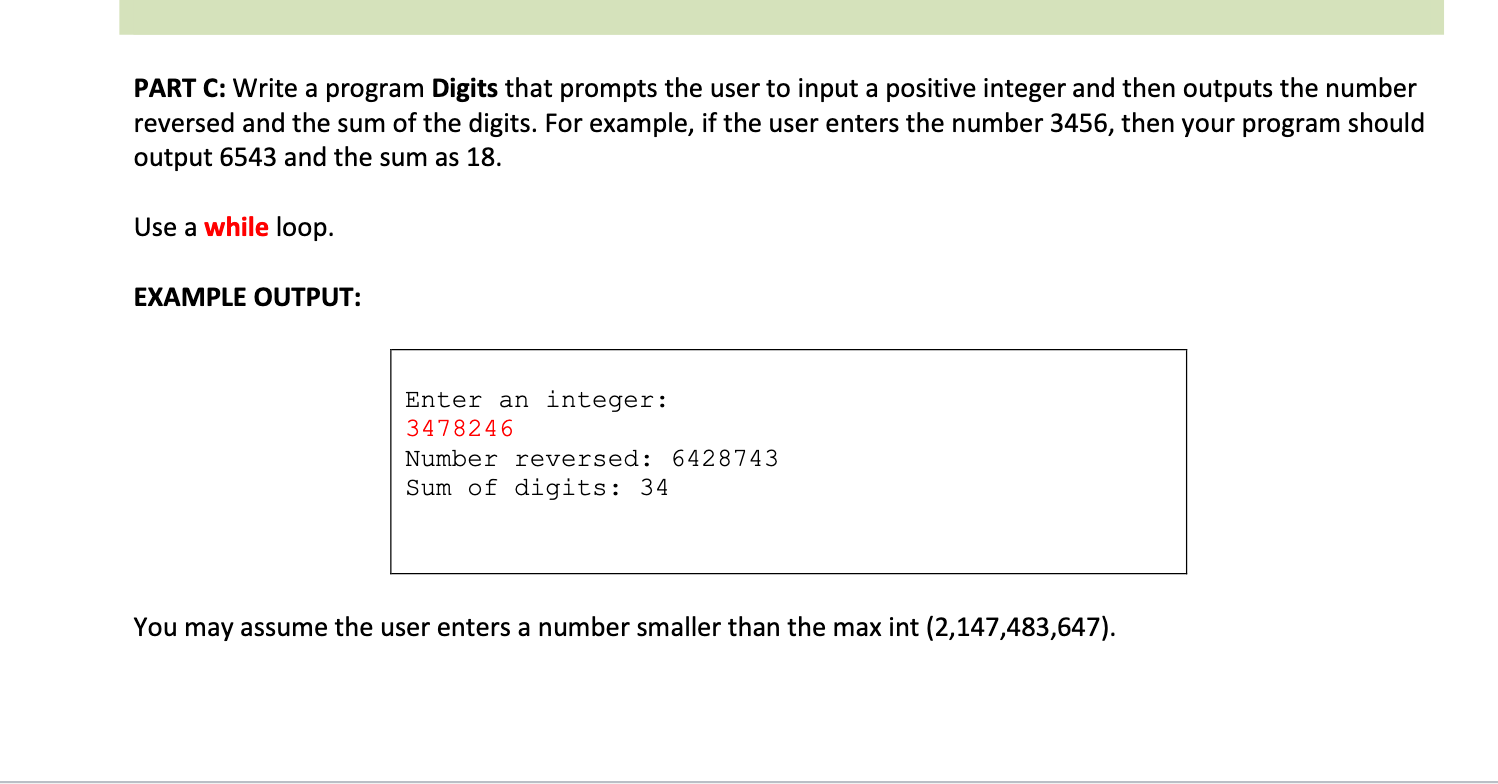# PART C: Write a program Digits that prompts the user to input a positive integer and then outputs the numberreversed and the sum of the digits. For example, if the user enters the number 3456, then your program shouldoutput 6543 and the sum as 18.Use a while loop.EXAMPLE OUTPUT:Enter an integer:3478246Number reversed: 6428743Sum of digits: 34You may assume the user enters a number smaller than the max int (2,147,483,647).

Question
83 views

Javahelp_outlineImage TranscriptionclosePART C: Write a program Digits that prompts the user to input a positive integer and then outputs the number reversed and the sum of the digits. For example, if the user enters the number 3456, then your program should output 6543 and the sum as 18. Use a while loop. EXAMPLE OUTPUT: Enter an integer: 3478246 Number reversed: 6428743 Sum of digits: 34 You may assume the user enters a number smaller than the max int (2,147,483,647). fullscreen
check_circle

Step 1

The below given java program will obey the following rubrics:

• Declaring main class.
• In the main method declaring some required variable.
• Displaying message to the user to enter the integer.
• Reading inputs from the user.
• Using a while loop to reverse the given number and to store the sum of the variable.
• In the while loop, taking the user entered number and processing the loop until the value of num becomes 0.
• Consider that the user entered number is 3478246. Now in the while loop, first step is to store the remainder of the given digit by dividing it with 10. So, here the remainder is 6 which is store in the digit variable. Now storing the value of digit variable in the reverse variable, by multiplying the initial value of reverse(0) value by 10. After this the next step is to divide the complete number by 10. So, here the result is taken in int i.e., 347824. So, our new number is 347824 storing this number in the num variable and adding the digits and storing the results in sum variable.
• Processing the above steps until the num becomes zero.
• At last after the while loop completes its processing then, displaying the reversed number to the user and showing the sum of the entered digits to user.

Step 2

Program code:

import java.util.Scanner;

//main class

public class Digits

{

//main method

public static void main(String[] args)

{

//declaring variables

long num, reversed = 0;

//instantiating Scanner class

Scanner sc = new Scanner(System.in);

//displaying message to user

System.out.println("Enter an integer: ");

num = sc.nextLong();

//declaring and initializing sum variable to store the sum

long sum = 0;

//while loop

while(num != 0)

{

//dividing the entered number by 10 and storing the remainder in the digit

long digit = ...

### Want to see the full answer?

See Solution

#### Want to see this answer and more?

Solutions are written by subject experts who are available 24/7. Questions are typically answered within 1 hour.*

See Solution
*Response times may vary by subject and question.
Tagged in